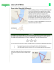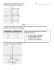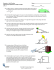# File

## Transcription

File
```unit 9 lesson 5.notebook
May 21, 2015
Warm Up (Exam Review)
Unit 9 Lesson 4 HW Answers
Using y = x2 + 2x + 3, 1. 120.7 ft
a. find the zeros
2. 65.40
b. find the y­intercept
3. 10.5 ft
c. determine the domain & range
4. 1427.2 ft
d. find the axis of symmetry
5. a: tan A = d/120
e. find the vertex
b. 51.30
f. graph
c. 590
6. 7. May 20­10:47 AM
Law of Sines
If ABC is a triangle with sides a, b, and c, then
May 20­10:49 AM
For the triangle in the figure, A=30˚, B=45˚, and a= 32. Find the remaining angles and sides
C
C
32
a
b
300
.
A
A
c
May 20­2:22 PM
450
B
B
May 20­2:27 PM
Ex 2) C = 102.30, B = 28.70, and b = 27.4 feet. Find the remaining angles and sides.
Because of prevailing winds, a tree grew so that it was leaning 6˚ from the vertical. At a point 30 meters from the tree, the angle of elevation to the top of the tree is 22˚. Find the height h of the tree.
May 20­2:29 PM
May 20­2:30 PM
1
unit 9 lesson 5.notebook
May 21, 2015
Practice
Ex 4) A pole tilts toward the sun at a 980 angle from the vertical, and it casts a 22­foot shadow. The angle of elevation from the tip of the shadow to the top of the pole is 430. How tall is the pole?
3. A = 360, a = 8, b = 5
16.70, a = 21.6
4. A = 102.40, C =
5. A = 830, C = 54.60, c = 18.1
16
6. A = 1100, a = 48, b =
7. Because of prevailing winds, a tree grew so that it was
leaning 40 from the vertical. At a point 35 meters from the
tree, the angle of elevation to the top of the tree is 230. Find the height (h) of the tree.
8. A flagpole at a right angle to the horizontal is located on
a slope that makes an angle of elevation of 120 with the
horizontal. The flagpole’s shadow is 16 meters long and
points directly up the slope. The angle of elevation from
the tip of the shadow to the sun is 200. Find the height of
the flagpole. May 20­2:31 PM
May 20­2:32 PM
Find the remaining angles and side of triangle ABC.
If you are given three sides (SSS), or two sides and their included angle (SAS), none of the ratios in the Law of Sines would be complete. In such cases, you can use the Law of Cosines.
16
800
12
May 20­2:33 PM
Example 2: Solve the triangle if A = 950, b = 12 and c = 15.
May 20­2:34 PM
Practice
1. 2. A = 1350, b = 4, c = 9
15
300
30
3. To approximate the length <javascript:de
('/Glossary/glossaryterm.aspx?word=Length',%20500,
20500);> of a lake, a surveyor starts at one end of the lake and
walks 245 yards. He then turns 110º and walks 270 yards until he
arrives at the other end of the lake. Approximately how long is
the lake?
May 20­2:35 PM
4. You are heading to Beech Mountain for a ski trip.
Unfortunately, state road 105 in North Carolina is blocked off
due to a chemical spill. You have to get to Tynecastle Highway
which leads to the resort at which you are staying. NC­105
would get you to Tynecastle Hwy in 12.8 miles. The detour
begins with a 18 veer off onto a road that runs through the local
city. After 6 miles, there is another turn <javascript:de
('/Glossary/glossaryterm.aspx?word=Turn',%20500,%20500);>
detour are straight, how many extra miles are you traveling to
May 20­2:35 PM
2
unit 9 lesson 5.notebook
May 21, 2015
May 21­10:13 AM
3
```

### Review12.1-12.6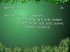### Worksheet - Multi-Step Trigonometry Problem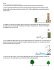### 7.6 Solving Right Triangle Problems.notebook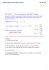### MCR3U - 4.2 - Applications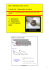### installation of an angle board * * * thermopian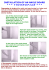### Student's worksheet - Eratosthenes Experiment 2014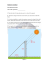### Wkst- Law of Sines-Area of Triangle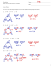### File - HHS Pre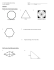### Final Review Finally - Mr. Mayer AfterMath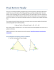### Trig Applications ws day 2 geo 14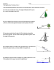### Torque/Cross Product Worksheet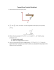### 12.1 Law of Sines - Pre